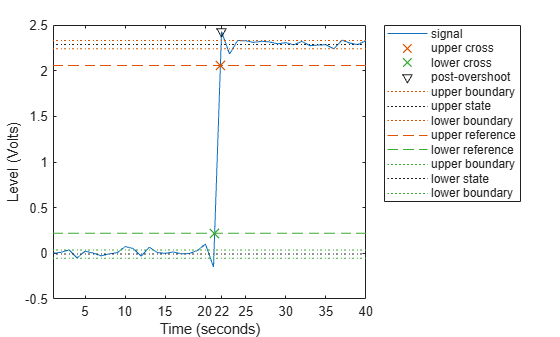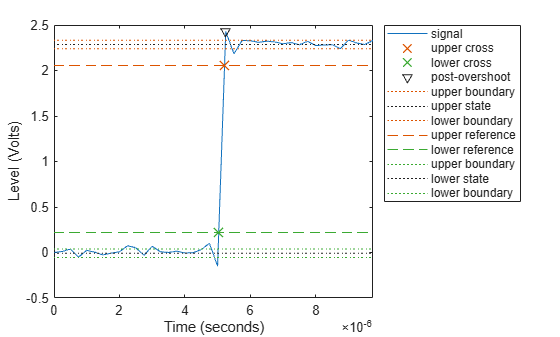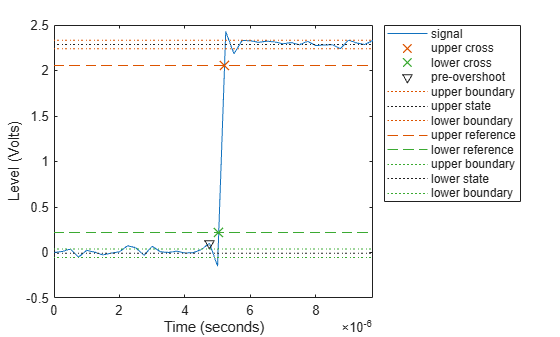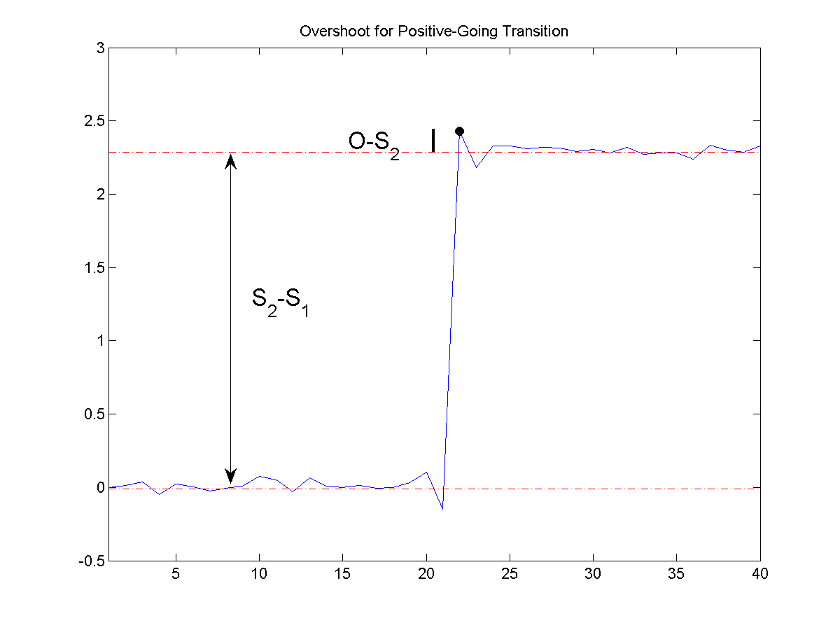Documentation

# overshoot

Overshoot metrics of bilevel waveform transitions

## Syntax

```OS = overshoot(X) OS = overshoot(X,FS) OS = overshoot(X,T) [OS,OSLEV,OSINST] = overshoot(...) [...] = overshoot(...,Name,Value) overshoot(...) ```

## Description

`OS = overshoot(X)` returns the greatest absolute deviations larger than the final state levels of each transition in the bilevel waveform, `X`. The overshoots, `OS`, are expressed as a percentage of the difference between the state levels. The length of `OS` corresponds to the number of transitions detected in the input signal. The sample instants in `X` correspond to the vector indices. To determine the transitions, `overshoot` estimates the state levels of the input waveform by a histogram method. `overshoot` identifies all intervals which cross the upper-state boundary of the low state and the lower-state boundary of the high state. The low-state and high-state boundaries are expressed as the state level plus or minus a multiple of the difference between the state levels. See State-Level Tolerances.

`OS = overshoot(X,FS)` specifies the sample rate in hertz. The sample rate determines the sample instants corresponding to the elements in `X`. The first sample instant in `X` corresponds to t=0.

`OS = overshoot(X,T)` specifies the sample instants, `T`, as a vector with the same number of elements as `X`.

```[OS,OSLEV,OSINST] = overshoot(...)``` returns the levels, `OSLEV`, and sample instants,`OSINST`, of the overshoots for each transition.

`[...] = overshoot(...,Name,Value)` returns the greatest deviations larger than the final state level with additional options specified by one or more `Name,Value` pair arguments.

`overshoot(...)` plots the bilevel waveform and marks the location of the overshoot of each transition as well as the lower and upper reference-level instants and the associated reference levels. The state levels and associated lower and upper-state boundaries are also plotted.

## Input Arguments

 `X` Bilevel waveform. `X` is a real-valued row or column vector. `FS` Sample rate in hertz. `T` Vector of sample instants. The length of `T` must equal the length of the bilevel waveform, `X`.

### Name-Value Pair Arguments

 `'PercentReferenceLevels'` Reference levels as a percentage of the waveform amplitude. The lower-state level is defined to be 0 percent. The upper-state level is defined to be 100 percent. The value of `'PercentReferenceLevels'` is a two-element real row vector whose elements correspond to the lower and upper percent reference levels. Default: `[10 90]` `'Region'` Specifies the region over which to compute the overshoot. Valid values for `'Region'` are `'Preshoot'` or `'Postshoot'`. If you specify `'Preshoot'`, the end of the pretransition aberration region is defined as the last instant where the signal exits the first state. If you specify `'Postshoot'`, the start of the posttransition aberration region is defined as the instant when the signal enters the second state. Default: `'Postshoot'` `'SeekFactor'` Aberration region duration. Specifies the duration of the region over which to compute the overshoot for each transition as a multiple of the corresponding transition duration. If the edge of the waveform is reached, or a complete intervening transition is detected before the duration aberration region duration elapses, the duration is truncated to the edge of the waveform or the start of the intervening transition. Default: `3` `'StateLevels'` Lower and upper state levels. Specifies the levels to use for the lower and upper state levels as a two-element real row vector whose first and second elements correspond to the lower and upper state levels of the input waveform. `'Tolerance'` Specifies the tolerance that the initial and final levels of each transition must be within the respective state levels. The `'Tolerance'` value is a scalar expressed as the percentage of the difference between the upper and lower state levels. Default: `2`

## Output Arguments

 `OS` Overshoots expressed as a percentage of the state levels. The overshoot percentages are computed based on the greatest deviation from the final state level in each transition. By default overshoots are computed for posttransition aberration regions. See Overshoot. `OSLEV` Level of the pretransition or posttransition overshoot. `OSINST` Sample instants of pretransition or posttransition overshoots. If you specify the sample rate or sampling instants, the overshoot instants are in seconds. If you do not specify the sample rate or sampling instants, the overshoot instants are the indices of the input vector.

## Examples

collapse all

Determine the maximum percent overshoot relative to the high-state level in a 2.3 V clock waveform.

Load the 2.3 V clock data. Determine the maximum percent overshoot of the transition. Determine also the level and sample instant of the overshoot. In this example, the maximum overshoot in the posttransition region occurs near index 22.

```load('transitionex.mat','x') [oo,lv,nst] = overshoot(x)```
```oo = 6.1798 ```
```lv = 2.4276 ```
```nst = 22 ```

Plot the waveform. Annotate the overshoot and the corresponding sample instant.

```overshoot(x); ax = gca; ax.XTick = sort([ax.XTick nst]);```Determine the maximum percent overshoot relative to the high-state level, the level of the overshoot, and the sample instant in a 2.3 V clock waveform.

Load the 2.3 V clock data with sampling instants. The clock data are sampled at 4 MHz.

`load('transitionex.mat','x','t')`

Determine the maximum percent overshoot, the level of the overshoot in volts, and the time instant where the maximum overshoot occurs. Plot the result.

`[os,oslev,osinst] = overshoot(x,t)`
```os = 6.1798 ```
```oslev = 2.4276 ```
```osinst = 5.2500e-06 ```
`overshoot(x,t);`Determine the maximum percent overshoot relative to the low-state level, the level of the overshoot, and the sample instant in a 2.3 V clock waveform. Specify the `'Region'` as `'Preshoot'` to output pretransition metrics.

Load the 2.3 V clock data with sampling instants. The clock data are sampled at 4 MHz.

`load('transitionex.mat','x','t')`

Determine the maximum percent overshoot, the level of the overshoot in volts, and the sampling instant where the maximum overshoot occurs. Plot the result.

`[os,oslev,osinst] = overshoot(x,t,'Region','Preshoot')`
```os = 4.8050 ```
```oslev = 0.1020 ```
```osinst = 4.7500e-06 ```
`overshoot(x,t,'Region','Preshoot');`collapse all

### Overshoot

For a positive-going (positive-polarity) pulse, overshoot expressed as a percentage is

`$100\frac{\left(O-{S}_{2}\right)}{\left({S}_{2}-{S}_{1}\right)}$`

where O is the maximum deviation greater the high-state level, S2 is the high state, and S1 is the low state.

For a negative-going (negative-polarity) pulse, overshoot expressed as a percentage is

`$100\frac{\left(O-{S}_{1}\right)}{\left({S}_{2}-{S}_{1}\right)}$`

The following figure illustrates the calculation of overshoot for a positive-going transition.The red dashed lines indicate the estimated state levels. The double-sided black arrow depicts the difference between the high and low-state levels. The solid black line indicates the difference between the overshoot value and the high-state level.

### State-Level Tolerances

Each state level can have associated lower- and upper-state boundaries. These state boundaries are defined as the state level plus or minus a scalar multiple of the difference between the high state and the low state. To provide a useful tolerance region, the scalar is typically a small number such as 2/100 or 3/100. In general, theregion for the low state is defined as

``

whereis the low-state level andis the high-state level. Replace the first term in the equation withto obtain thetolerance region for the high state.

The following figure illustrates lower and upper 2% state boundaries (tolerance regions) for a positive-polarity bilevel waveform. The red dashed lines indicate the estimated state levels.## References

 IEEE® Standard on Transitions, Pulses, and Related Waveforms, IEEE Standard 181, 2003, pp. 15–17.